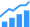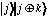# How To Program A Quantum Computer

There are infinite possibilities of one-qubit operations, but so far none have been implemented in hardware.Quantum computers have captured the attention of the computer science world because they are faster than classical computers for some problems. Spend any time reading about quantum computing technology, and you’ll see that statement over and over again. But what does it actually mean, given that classical computing is a mature, highly optimized technology and quantum computers are in their infancy?

The phrase “quantum speedup” is used to describe a wide range of situations, in which the advantage quantum computers offer might be quite large, relatively small, or non-existent. At one extreme, we find “strong” quantum computing algorithms. These are the algorithms that started it all, that convinced the community that quantum computing was worth pursuing. Grover’s search algorithm, for example, is provably faster than any known or potential classical search algorithm. In the best classical search algorithms, the time required grows at the same rate as the number of items, while the time required by Grover’s algorithm increases only as the square root of the number of items. Similarly, Shor’s algorithm for factoring large numbers is faster than any known classical algorithm, with the processing time increasing as (log N)³, where N is the number being factored and log N is its length in bits. A quantum computer using these algorithms will require fewer computational steps than a classical computer solving the same problems.

Shor’s algorithm, Grover’s algorithm, and others like them were originally conceived using a model of quantum computation that resembles conventional gate-based logic. Theoreticians have shown that any reversible classical gate can be emulated by some combination of operations on a single qubit plus the CNOT quantum gate. CNOT is a two-qubit operation in which the state of the first qubit controls the state of the second: the gate transforms the initial statetowhere the ⊕ indicates modulo 2 addition.

There are infinitely many potential one-qubit operations. While only some of these operations will be available in any given physical system, the conclusion of all this theory is that it is possible to design a universal, general purpose quantum computer that can emulate any classical computation.

However, no such computer has yet been implemented in hardware. As discussed elsewhere in this series, most proposed qubit systems have struggled to assemble more than a handful of interacting qubits. Intel’s 4004, the first conventional microprocessor, in contrast, contained 2,300 transistors. In 2014, state of the art commercial chips have 5 billion to 7 billion transistors. A quantum computer capable of implementing Shor’s algorithm would require (log N)³ qubits: trillions, for the large numbers of interest in cryptography. An actual working computer that can implement Shor’s algorithm for large numbers will require an increase in scale comparable to the jump from individual transistors up to or beyond current integrated circuit technology. Even under the most optimistic projections, such a computer lies many years in the future. Indeed, it’s not clear whether it can be built at all.

Error correction and scaling
Among many other challenges, large quantum computers will incur substantial overhead due to error correction mechanisms. Individual qubit states are very fragile, prone to decoherence and disruption by environmental noise. They are unlikely to remain stable for more than a few milliseconds. A functional quantum computer will need to use some form of error correction to “reset” the quantum state after such disruptions.

Error correction is a major topic in its own right, far beyond the scope of this article. Briefly, error correction schemes use additional classical and/or quantum circuit elements to detect errors as they occur. This is more difficult than it might sound: remember that measuring a qubit’s quantum state collapses the superposition of states that is so essential to quantum computing. Instead, the system design must measure the entire superposition — multiple qubits at once — and reinitialize the system as a whole if necessary. Depending on the chosen error correction scheme, a single logical qubit might be composed of anywhere from a few dozen to a few thousand physical qubits.

No proposed qubit design has achieved anything approaching the device densities that classical integrated circuits take for granted. Combine low device densities, very large numbers of physical qubits, and complex classical control and monitoring circuits, and it becomes clear that the performance of quantum computers may be limited by prosaic concerns such as signal propagation time and the speed of the classical control elements, rather than the speed of the quantum elements themselves.

The first commercial applications of quantum computers are likely to have much more modest ambitions, and the advantages of quantum computation will be much less obvious. In some cases, a quantum algorithm may be faster than a particular group of classical algorithms, even if it doesn’t surpass the best known classical algorithms. Or quantum hardware might be faster than classical hardware for specific types of problems. For example, ranking a large index is a reasonably tractable problem for classical algorithms. The best algorithms scale as either N or N log N, where N is the size of the index. However, ranking indexes to very large databases, such as the Web, still takes weeks. Even if quantum computation achieves only modest improvements, it may still be worth pursuing for some problems.

Simulations of quantum systems may fall into this category, too, as may some types of optimization problems. This is the domain that D-Wave Systems has chosen to explore: D-Wave chief scientist Eric Ladizinsky freely concedes that the company’s system is not a general purpose computer. Rather, the D-Wave system is designed to apply quantum annealing to optimization problems that can be defined in terms of an array of interacting spins. Though not universal, this “Ising glass” model applies to an enormous number of problems of practical interest, from obvious applications in phase transformations and the movement of atoms to fields as diverse as neurobiology and sociology. More precisely, these problems can be expressed in the form:For a given set of parameters h, and K, the task is to find the optimal solution vector s that minimizes the value E, the overall system “energy.” Adiabatic quantum optimization begins by preparing a qubit array in the ground state, described by the Hamiltonian HB. This initial state should be one that is easy to create, but otherwise the choice is arbitrary.

By slowly advancing the parameter k from 0 to 1, the system Hamiltonian evolves toward one representing the problem being investigated, HP.Once the target Hamiltonian has been reached, measuring the resulting quantum state gives the solution. For sufficiently small increments, the system remains in the ground state throughout. As a result, it is relatively stable in the face of environmental noise.

One challenge for all optimization algorithms, classical or quantum, is that the algorithm may find a local minimum rather than the true lowest energy state. The element of randomness in quantum states allows quantum algorithms to potentially “tunnel” out of these local minima with some probability depending on the system design. It is also possible to run the algorithm many times, with many choices of initial parameters, in order to more thoroughly explore the set of potential solutions.

As the number of near-solutions increases, however, the increment k that must be used in order to remain in the ground state and maintain the system’s adiabatic condition becomes smaller. Ultimately, finding the true minimum of the system may require exponentially small increments and exponentially long times. In some circumstances, finding a local minimum may be a “good enough” solution. Still, there remains a great deal of debate about how much speedup the D-Wave approach actually offers, and whether the advantages are due to quantum effects or to other features of the system architecture.

Conclusions
As often happens with emerging technologies, the promise of quantum computing has leaped far ahead of the practical reality. Algorithms that will require many millions of qubits are paired with hardware that can support, at most, a few hundred. As was the case in the early years of the integrated circuit industry, the limitations of the hardware show how much work remains to be done. The promise of the algorithms shows why the effort is worth making.Sk Vader says:

“A quantum computer capable of implementing Shor’s algorithm would require (log N)³ qubits: trillions, for the large numbers of interest in cryptography.”

If (log N)³ = 10^12 (trillion) then N (the number of bits in the number being factored) > 4.3 x 10^9999. Given that the number of atoms in the observable universe is estimated to be 10^82 that is clearly a ridiculous assertion.Roto3 says:

A very good and well grounded discussion of quantum computing. What it is and what it is not. As a physicist, I find the approach taken very condensed (appreciated) and within the realm of reason (which many quantum computing articles are not). This is the first I’ve heard of trying to simulate a classical computer with qubits. It’s interesting, but likely to lead to the circular result that its not faster than classical. Its still possible that qubits may be configured to represent a very specific problem and measured repeatedly (starting from the initial state) until a solution of arbitrary accuracy is obtained. Essentially, the state dependent analog of the old analog computer differing in that arbitrary accuracy can be obtain which was not true for the old analog method. Such computation may be faster. A very narrow focus, but possibly useful. A quantum general purpose computer I do not expect to have advantage.JC says:

Actually, log N itself is the number of bits, not N (according to the Wikipedia article linked to for Shor’s algorithm). Then the number do make sense.kderbyshire says:

Ah, I see the problem. In some sources, N is the number being factored, in which case log N (binary) is the number of bits. In others, N is the number of bits. Inconsistent usage trips up unwary reader!

I’ll fix the article to clarify this point. Thank you for calling it to my attention.kderbyshire says:

The ability to implement classical algorithms in quantum computers is relevant to discussions of the universality of quantum computing, and thus to debates about what sort of problems quantum computers might be able to solve. That’s a big topic, well beyond the scope of this series. “Quantum Computation and Quantum Information,” by Nielsen and Chuang, is the standard introductory textbook and reasonably accessible to non-specialists.

The problem with repeated measurements is that, as you know, measuring a quantum system forces it to take on a single state, rather than maintaining a superposition of all states. In other words, measurement stops the computation, and it can’t be restarted easily. However, a group at University of Chicago recently demonstrated a promising technique for watching quantum systems evolve: http://semiengineering.com/watching-qubits-at-work/